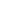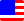Documents  >  Tutorial  >  Compound assignment
Compound assignment statement

It performs arithmetic operations and assignments at the same time.

Format

Lvalue

Assignment symbol

Expression

Semicolon

Example

Format

The format of the compound assignment statement are:

lvalue *= expression ;

lvalue /= expression ;

lvalue %= expression ;

lvalue += expression ;

lvalue -= expression ;

Each is equivalent to the following assignment statement.

lvalue = left * right ;

lvalue = left / right ;

lvalue = left % right ;

lvalue = left + right ;

lvalue = left - right ;

The lvalue is things that can be placed on the left side of the assignment symbol. In Rice, they are variables, fields , expressions that end with a setter , and expressions that end with an indexer operator.

Arithmetic operation is performed on the instances returned by the expressions on the left and right sides of the assignment symbol, and the lvalue is changed by the result.

If the lvalue ends with a variable or field, changes the address referenced by the lvalue.

If the lvalue ends with a setter, it is converted to a setter call.

If the lvalue ends with an indexer operator, changes an item of corresponding list class.

Lvalue

The lvalue is things that can be placed on the left side of the assignment symbol. In Rice, they are variables, fields, expressions that end with a setter, and expressions that end with an indexer operator.

The lvalue of the compound assignment statement is evaluated as an expression for arithmetic operations before the assignment and returns an instance. The returned instance must be an int, long, or real class.

Assignment symbol

Assignment symbols for compound assignment statement are:

*=

/=

%=

+=

-=

Each corresponds to an arithmetic operator. Arithmetic rules are the same as the corresponding operator.

The assignment rules are the same as the assignment statement.

Expression

The expression returns an instance for arithmetic operations. The returned instance must be an int, long, or real class.

Semicolon

The end of a compound assignment statement is represented by a semicolon.

Example

 1: class example 2: int var1; 3: open method void someMethod() 4: var1 = 10; 5: var1 += 10; // var1 is 20. 6: em 7: ec